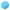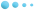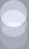HomeASP HostingWeb DesignRegister DomainSupportAbout UsFAQSupport :: FAQ##### Eval() and Execute() VBScript functions – how to use them in ASP
Microsoft has released 2 very useful functions with Microsoft Visual Basic Scripting Edition 5.0, namely Eval() and Execute() functions. We will compare those 2 functions and give examples of their use in this article.

The Eval() VBScript function simply evaluates an expression and returns the result. Let’s have a look at the following line of VBScript code:

Var1 = Var2

You can interpret this statement in 2 completely different ways. The first one is "the value of Var2 is assigned to Var1” and the second one is "Var1 is compared to Var2”. The Eval() VBScript function always uses the second interpretation and returns Boolean value - True or False. For example consider the following ASP code:

<%

Var1 = 1
Var2 = 2
Response.Write(Eval("Var1 = Var2 + 1")) ' Prints False
Response.Write(Var1) ' Prints 1, even after the Eval() function execution on the previous line
Response.Write(Eval("Var1 = Var2 - 1")) ' Prints True

%>

The Execute() VBScript function uses the first interpretation we talked about earlier, which actually evaluates the expression parameter. For example the following ASP code will print 5 in the browser:

<%

Var1 = 1
Var2 = 2
Execute("Var1 = Var2 + 3")
Response.Write (Var1) ' Prints 5
%>